Algebra II - Complex and Imaginary Numbers Review: Tutoring Solution Chapter Exam

Exam Instructions:

Choose your answers to the questions and click 'Next' to see the next set of questions. You can skip questions if you would like and come back to them later with the yellow "Go To First Skipped Question" button. When you have completed the practice exam, a green submit button will appear. Click it to see your results. Good luck!

Answered 0 of 25

Page 1

Question 1 1. What type of solution(s) would you get from the equation below?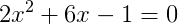Question 2 2. Which number is represented by the graph below?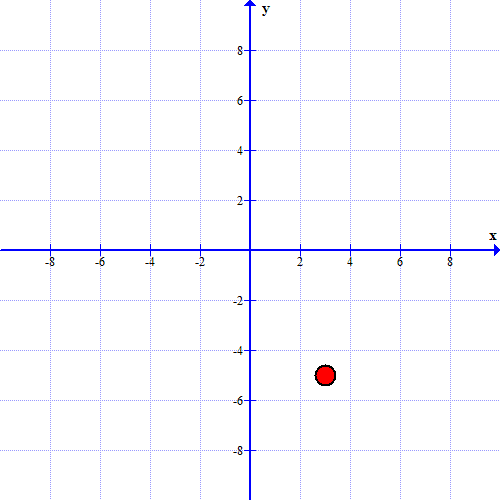Question 3 3. Simplify.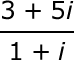Question 4 4. What is the most simplified form of the expression below?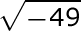Page 2

Question 6 6. Simplify.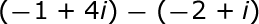Question 7 7. What is the simplified value of the expression below?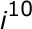Question 8 8. Simplify.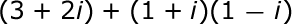Question 9 9. Simplify.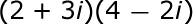Page 3

Question 11 11. Which number is represented by the graph below?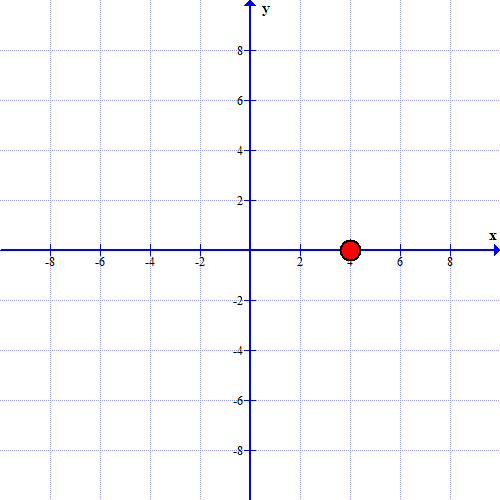Question 13 13. Which statement MUST be true about the equation below?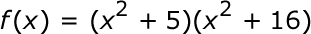Question 14 14. How many roots does the equation below have?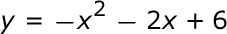Question 15 15. Simplify.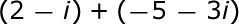Page 4

Question 16 16. What would be the first step in dividing the complex numbers below?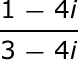Question 18 18. Find the roots of the equation below: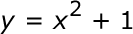Page 5

Question 21 21. Simplify.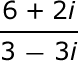Question 23 23. Solve the equation below: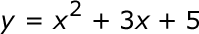Question 24 24. What is the complex conjugate of the expression below?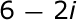Algebra II - Complex and Imaginary Numbers Review: Tutoring Solution Chapter Exam Instructions

Choose your answers to the questions and click 'Next' to see the next set of questions. You can skip questions if you would like and come back to them later with the yellow "Go To First Skipped Question" button. When you have completed the practice exam, a green submit button will appear. Click it to see your results. Good luck!

Support